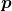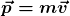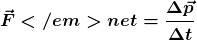# eqn

Definition of momentum\\

It is convenient to define a physical quantity called momentum, usually indicated by the symbol, as the product of its massby its velocity(1)The momentum is a vector quantity which has the same direction as the velocity of a body.

The unit of measurement of momentum in the SI system is the product of the units of mass and velocity and thus is the kg m/s.

A new form of the Second Newton’s Law\

Newton’s Second Law can be expressed in terms of change in momentum:

(2)which can be written in the more compact form

(3)